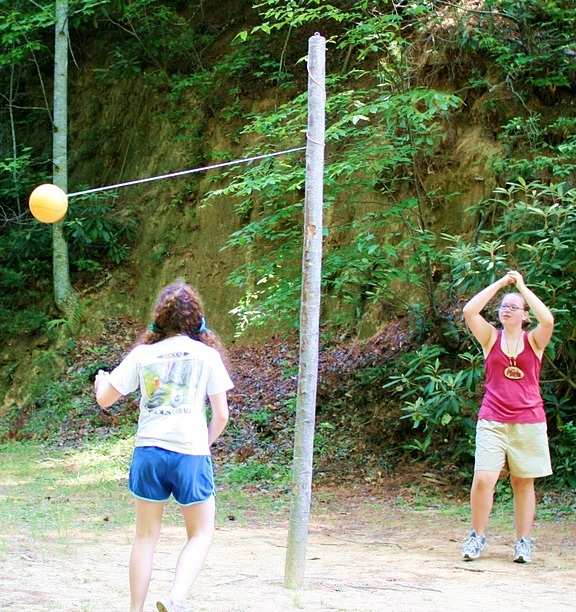# Re: Converse of Pythagorean Theorem

Forums Main Page Forums Main Page Geometry Converse of Pythagorean Theorem Re: Converse of Pythagorean Theorem

#529Anonymous
Inactive

One way construction workers can determine whether a piece of wood or pole, a tetherball pole for example, is perpendicular with the ground is by placing a 5 foot string exactly three feet from the ground, and placing it at some point on the pole to create a triangle–with the ground, pole portion, and string as the three sides. They then can measure up the pole to see if the remaining distance from the ground to the end of the string is exactly 4 feet, so as to create a 3, 4, 5 right triangle. If it is not exactly 4 feet, they know the triangle is not a right triangle, hence the pole is not perpendicular as shown in the pole below…